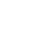# What is the ‘First Half Odd/Even Goals’ Market?

•First Half Odd/Even Goals

This market allows the punter to bet on whether there will be an odd number of goals, or an even number of goals scored in the 1st half.

If Swallows is playing Kaizer Chiefs, then the market options are:
1. SvK ODD NUMBER TOTAL GOALS
2. SvK EVEN NUMBER TOTAL GOALS

Therefore if the score at the end of the first half is 3-0, then this means there was an odd number of goals scored in the 1st half.  The SvK ODD NUMBER TOTAL GOALS option will be the winner.

If the score at the end of the first half is 1-1, then this means that there was an even number of goals scored in the first half (two goals in total).  Therefore the SvK EVEN NUMBER TOTAL GOALS option is the winner.

If the score ends at 0-0 at the end of the first half, the SvK EVEN NUMBER TOTAL GOALS will be considered the winner.  Therefore ‘no goals’ is equal to an ‘even number’.

To find this market on the Hollywood website or mobile app, look for the match and the scroll to the FIRST HALF ODD / EVEN GOALS market.